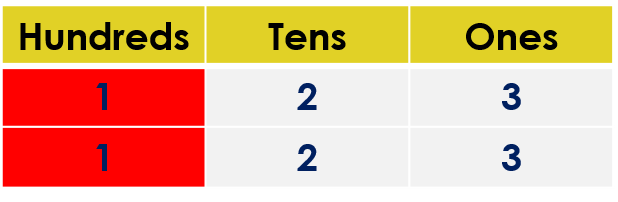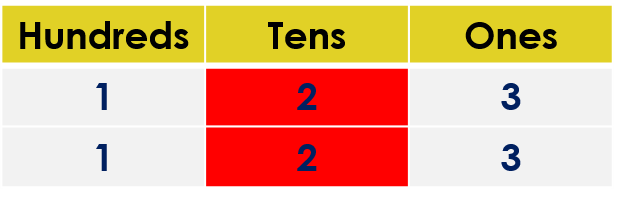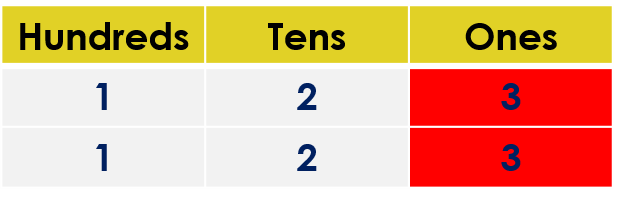1
visibility
Fill in the blank space with the correct sign. Use either <, > or =.
123  \$latex \framebox[0.5in]{}\$  123

The correct answer is "=".

It is easy!

• Compare the hundreds digits.
• If the hundreds digits are the same, then compare the tens digits.
• If tens digits are the same, then compare the ones digits.

Solution:

Observe that both numbers have three digits.

123 ____ 123

Comparing the hundreds digits:We observe that both digits are the same.

Comparing the tens digits:We observe that both digits are the same.

Comparing the ones digits:We observe that both digits are the same.

So, 123 is equal to 123.

That is, 123 = 123.